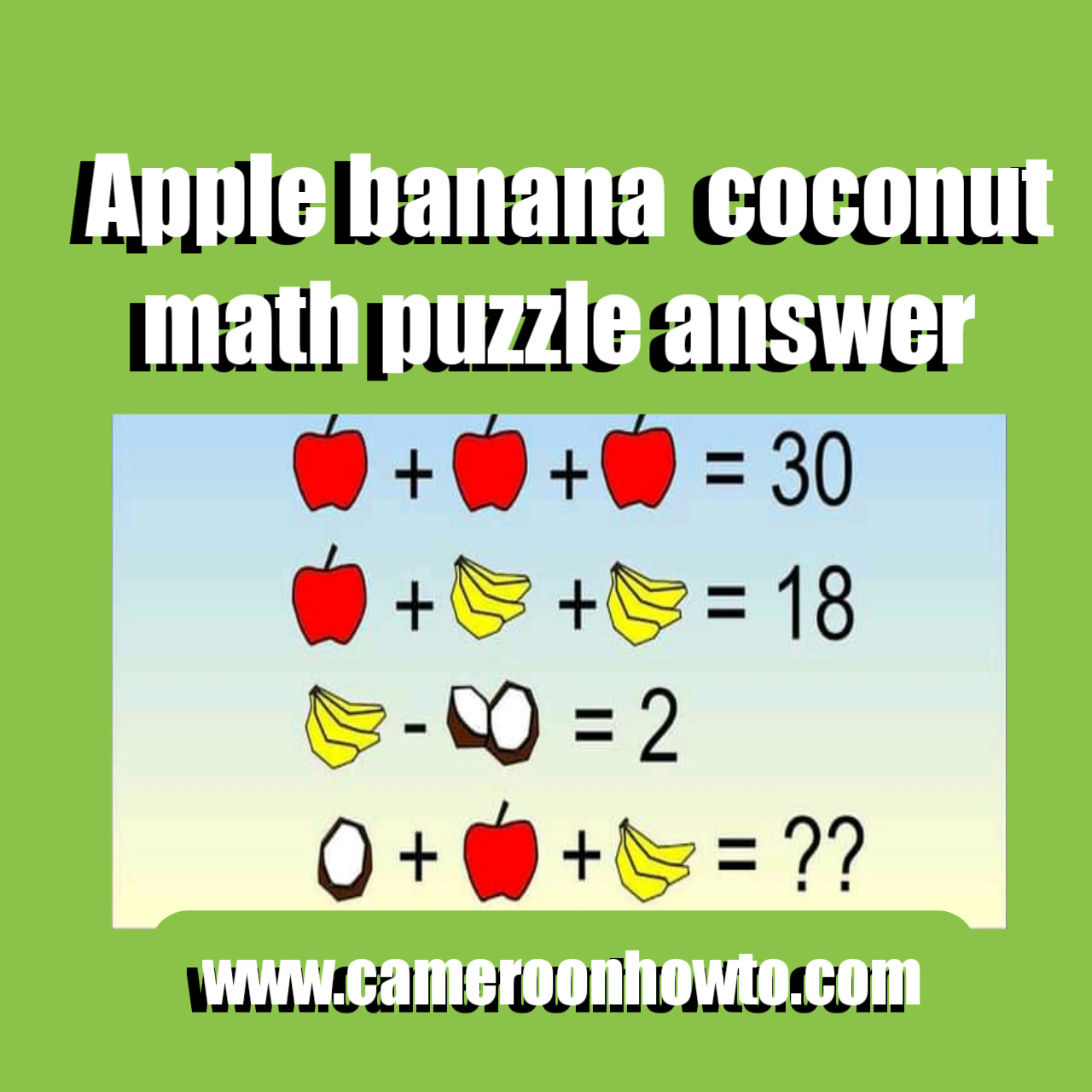0

# Apple banana  coconut math puzzle answerApple banana  coconut math puzzle answer

This is one of those fruit math  puzzles that keeps many people scratching their heads on the internet, especially on Facebook.  When the Puzzle was published on Facebook, many people solved it and had different opinions and answers. Today I will give you the correct answer to the puzzle with solving detail.

## Half Coconut + apple + bananas = ?

The answer would be 14 not 16, the trick in the fruit math Puzzle is found in the last equation. Check solving below, per attention to the bounces of Bananas.

Here's the puzzle extraction:

If

Apple + Apple + Apple = 30,

Apple + 4bananas + 4bananas = 18

4 bananas - 2half coconut = 2

then,

Half coconut + apple + Three bananas = ?

Many game lovers like solving math puzzles suduku, quizzes like this one to refresh their brain.

Due to the Corona various pandemic, many people isolate themselves because of lockdowns and to avoid contracting or spreading the deadly disease.

Isolation can lead to boredom,  one way to refresh the brain, relax and get busy is  by solving Math puzzles and Quizzes.

I will solve each equation and then substitute answers in the final equation and simplify.

Equation one

3apples =30

Implies Apple = 30÷3, Apple = 10.

Equation two

10+ 8bananas = 18

Implies 8bananas = 18 minus 10

8 bananas = 8

Banana = 8÷ 8

Banana = 1

Equation three

4 - 2halfs coconut= 2

-2 halfs coconut = -2

2 halfs coconut = 2

Half Coconut = 1

Therefore, the value of the variables are as Thus:

Apple= 10

Banana = 1

Half Coconut = 1

Final equation

I will substitute the values of the variables above in the final equation below and simplify.

Half Coconut + apple + bananas = ?

1 + 10 + 3 =?

14 = ?

So the correct answer to the tricky  math Puzzle Half Coconut + apple + bananas =? Is

= 14.

Do you have another way to arrive at this Answer? Share with us.

Don't forget to test your friends, family members and loved ones by sharing the puzzle.

Tags